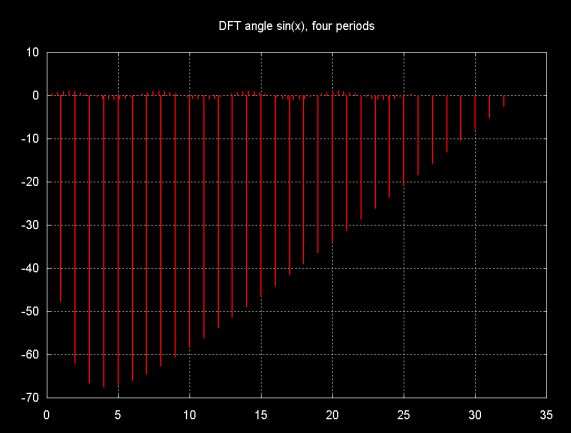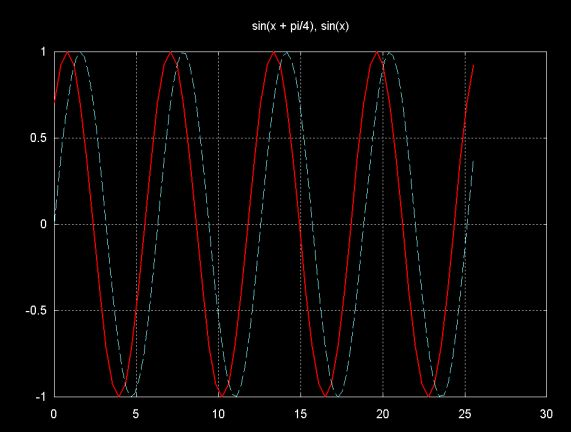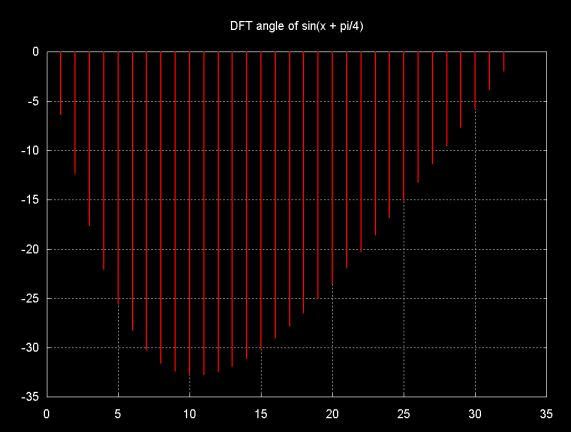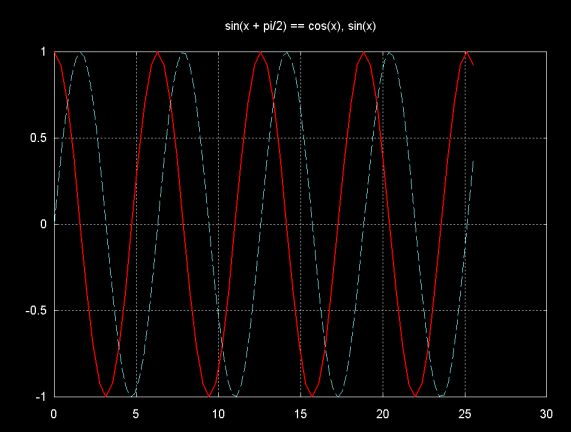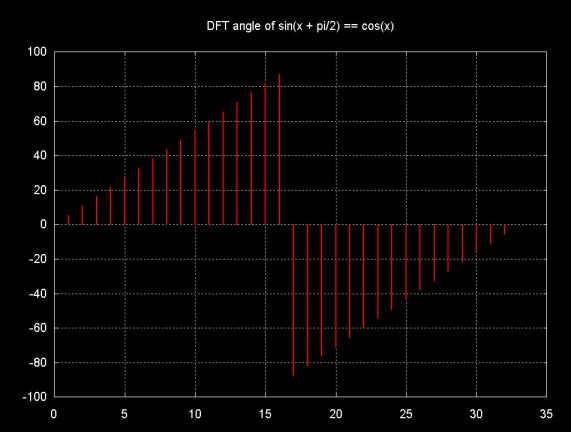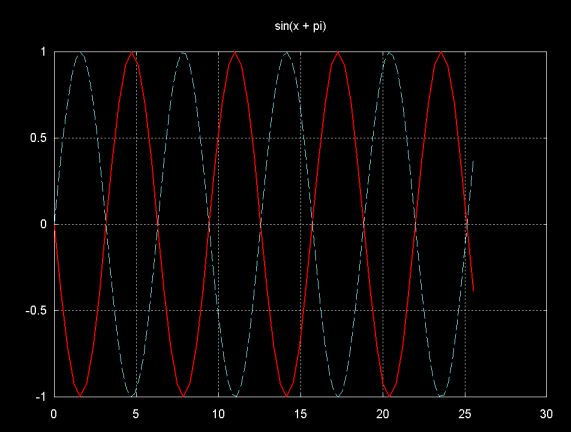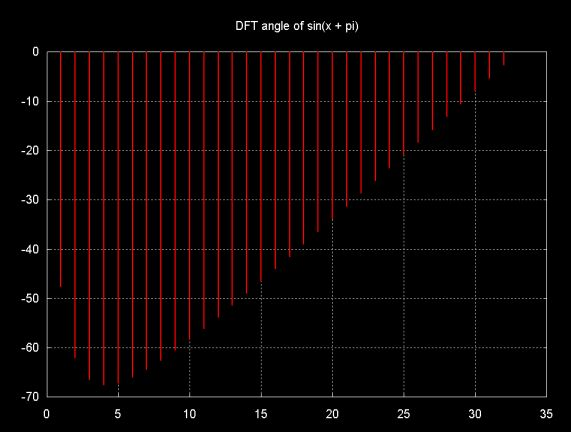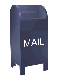DFT Angle

The result of the DFT is a complex number. This can be plotted either as a magnitued, as I've done on this web page, or as an angle. The magnitude is the hypotenuse of the triangle formed by the real and imaginary parts of complex number (where the imaginary part is plotted on the y-axis and the real part is plotted on the x-axis). The angle is

angleradians = arctan( imag / real )